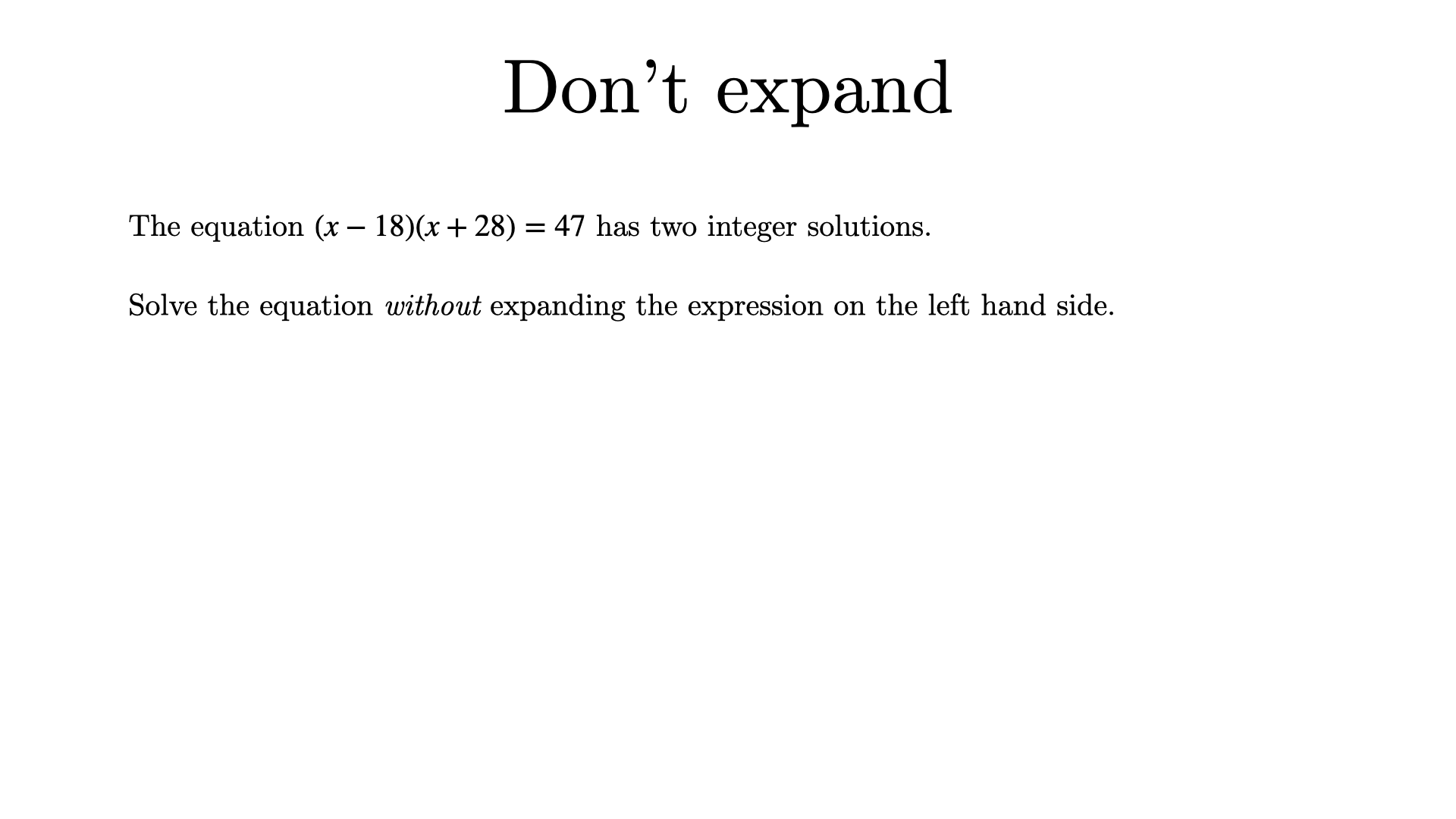Solution

Given that the equation has integer solutions, the two factors $$(x-18)$$ and $$(x+28)$$ must both be integers. The product of these must be 47. Note also that the latter factor is greater than the former. This is only possible if either $$(x-18)=1$$ and $$(x+28)=47$$, or if $$(x-18)=-47$$ and $$(x+28)=-1$$. Hence the two solutions are $$x=19$$ and $$x=-29$$.